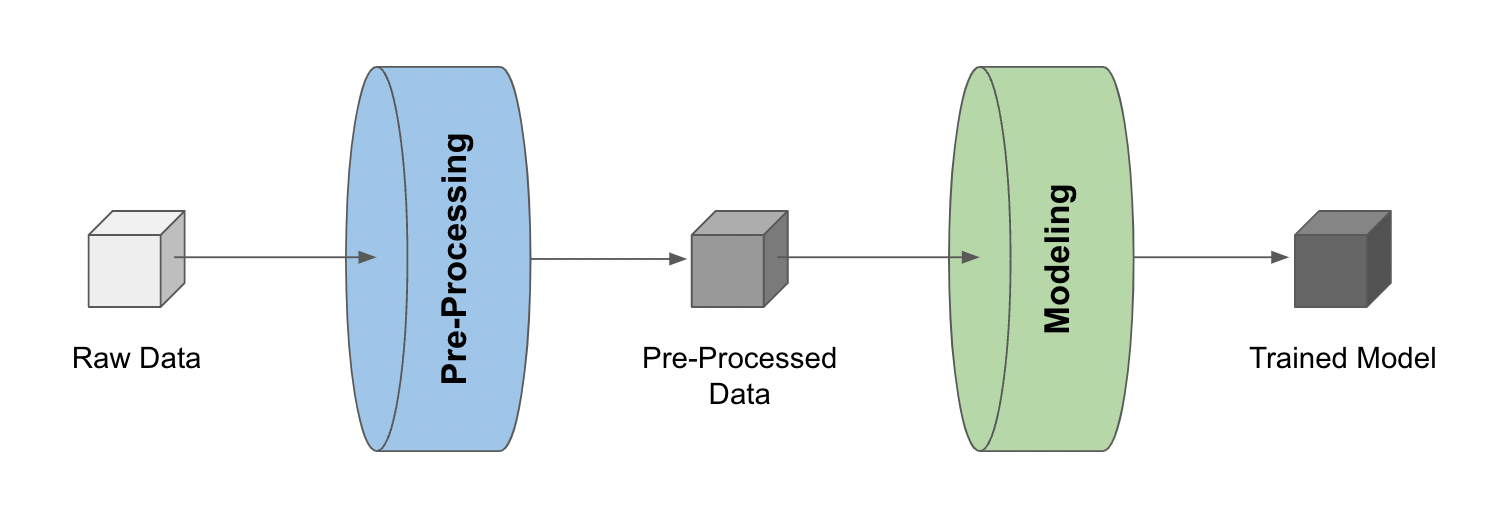# Building a Pipeline from Development Code¶

To generate a pipeline from development code, first ensure variable(s) of interest have been stored as LineaPy artifacts:

``````lineapy.save(<VARIABLE-NAME>, "<artifact_name>")
``````

Then, building a pipeline reduces to “stitching” these artifacts, like so:

``````lineapy.to_pipeline(
pipeline_name="<pipeline_name>",
artifacts=["<artifact_name>", ...],
dependencies={
"<artifact_name>": {"<artifact_name>", ...},
...
},
output_dir="<output_dirpath>",
framework="<framework_name>",
)
``````

where

• `pipeline_name` is the name of the pipeline

• `artifacts` is the list of artifact names to be used for the pipeline

• `dependencies` is the dependency graph among artifacts

• If artifact A depends on artifacts B and C, then the graph is specified as `{ A: { B, C } }`

• If A depends on B and B depends on C, then the graph is specified as `{ A: { B }, B: { C } }`

• `output_dir` is the location to put the files for running the pipeline

• `framework` is the name of orchestration framework to use

• LineaPy currently supports `"AIRFLOW"`, `"ARGO"`, `"KUBEFLOW"`, `"RAY"`, and `"SCRIPT"`

• If `"SCRIPT"`, it will generate files that can run the pipeline as a Python script

• Otherwise files corresponding to the orchestration framework selected will be generated

Info

Check `lineapy.to_pipeline()` for more detailed API information.

## Airflow Example¶

For example, consider a simple pipeline that 1) pre-processes raw data and 2) trains a model with the pre-processed data.With the pre-processed data and the trained model stored as LineaPy artifacts (which can be done during development sessions), building an Airflow pipeline becomes as simple as the following:

``````lineapy.to_pipeline(
pipeline_name="iris_pipeline",
artifacts=["iris_preprocessed", "iris_model"],
dependencies={"iris_model": {"iris_preprocessed"}},
output_dir="~/airflow/dags/",
framework="AIRFLOW",
)
``````

where `{"iris_model": {"iris_preprocessed"}}` is a way to indicate that the `"iris_model"` artifact depends on the `"iris_preprocessed"` artifact.

Running this creates files (under `output_dir`) that can be used to execute the pipeline as an Airflow DAG, including:

• `<pipeline_name>_module.py`: Contains the artifact code refactored and packaged as function(s)

• `<pipeline_name>_dag.py`: Uses the packaged function(s) to define the framework-specific pipeline

• `<pipeline_name>_requirements.txt`: Lists any package dependencies for running the pipeline

• `<pipeline_name>_Dockerfile`: Contains commands to set up the environment to run the pipeline

where `<pipeline_name>` is `iris_pipeline` in the current example.

Output File Examples
``````import pandas as pd
from sklearn.linear_model import LinearRegression

def get_iris_preprocessed():
url = "https://raw.githubusercontent.com/LineaLabs/lineapy/main/examples/tutorials/data/iris.csv"
color_map = {"Setosa": "green", "Versicolor": "blue", "Virginica": "red"}
df["variety_color"] = df["variety"].map(color_map)
df["d_versicolor"] = df["variety"].apply(lambda x: 1 if x == "Versicolor" else 0)
df["d_virginica"] = df["variety"].apply(lambda x: 1 if x == "Virginica" else 0)
return df

def get_iris_model(df):
mod = LinearRegression()
mod.fit(
X=df[["petal.width", "d_versicolor", "d_virginica"]],
y=df["sepal.width"],
)
return mod

def run_session_including_iris_preprocessed():
# Given multiple artifacts, we need to save each right after
# its calculation to protect from any irrelevant downstream
# mutations (e.g., inside other artifact calculations)
import copy

artifacts = dict()
df = get_iris_preprocessed()
artifacts["iris_preprocessed"] = copy.deepcopy(df)
mod = get_iris_model(df)
artifacts["iris_model"] = copy.deepcopy(mod)
return artifacts

def run_all_sessions():
artifacts = dict()
artifacts.update(run_session_including_iris_preprocessed())
return artifacts

if __name__ == "__main__":
# Edit this section to customize the behavior of artifacts
artifacts = run_all_sessions()
print(artifacts)
``````
``````import pathlib
import pickle

import iris_pipeline_module
from airflow import DAG
from airflow.operators.python_operator import PythonOperator
from airflow.utils.dates import days_ago

def dag_setup():
pickle_folder = pathlib.Path("/tmp").joinpath("iris_pipeline")
if not pickle_folder.exists():
pickle_folder.mkdir()

def dag_teardown():
pickle_files = (
pathlib.Path("/tmp").joinpath("iris_pipeline").glob("*.pickle")
)
for f in pickle_files:

df = iris_pipeline_module.get_iris_preprocessed()

pickle.dump(df, open("/tmp/iris_pipeline/variable_df.pickle", "wb"))

mod = iris_pipeline_module.get_iris_model(df)

pickle.dump(mod, open("/tmp/iris_pipeline/variable_mod.pickle", "wb"))

default_dag_args = {
"owner": "airflow",
"retries": 2,
"start_date": days_ago(1),
}

with DAG(
dag_id="iris_pipeline_dag",
schedule_interval="*/15 * * * *",
max_active_runs=1,
catchup=False,
default_args=default_dag_args,
) as dag:

setup = PythonOperator(
python_callable=dag_setup,
)

teardown = PythonOperator(
python_callable=dag_teardown,
)

iris_preprocessed = PythonOperator(
)

iris_model = PythonOperator(
)

iris_preprocessed >> iris_model

setup >> iris_preprocessed

iris_model >> teardown
``````
``````lineapy
pandas==1.3.5
matplotlib==3.5.1
scikit-learn==1.0.2
``````
``````FROM apache/airflow:latest-python3.10

RUN mkdir /tmp/installers
WORKDIR /tmp/installers

# copy all the requirements to run the current dag
COPY ./iris_pipeline_requirements.txt ./
# install the required libs
RUN pip install -r ./iris_pipeline_requirements.txt

WORKDIR /opt/airflow/dags
COPY . .

WORKDIR /opt/airflow

CMD [ "standalone" ]
``````

## Running Locally¶

With the pipeline files generated, we can quickly test running the pipeline locally. First, run the following command to build a Docker image:

``````docker build -t <image_name> . -f <pipeline_name>_Dockerfile
``````

where `<pipeline_name>_Dockerfile` is the name of the automatically generated Dockerfile and `<image_name>` is the image name of our choice.

We then stand up a container instance with the following command:

``````docker run -it -p 8080:8080 <image_name>
``````

For `framework="AIRFLOW"`, this will result in an Airflow instance with an executable DAG in it.